# 证明与计算(3): 二分决策图(Binary Decision Diagram, BDD)

## 0x01 布尔代数(Boolean algebra)

“这个过程是必然会发生的么？”stephen wolfram思考到。布尔代数的历史显示了一个从复杂问题中慢慢产生的简单的形式化思想，偶然间就被大规模使用了，流行了起来。

Most often what happens is that at some moment the ideas become relevant to technology, and quickly then go from curiosities to mainstream.

stephen wolfram的书《A New Kind of Science》收集了很多这种“simple formal idea”，其中有些已经流行，有很多还没有，其中会有下一个技术奇点么？

## 0x02 布尔函数(Boolean function)

• F:$\{0,1\}^k->\{0,1\}$

• Nulary：F()=0，也就是常量布尔函数
• Unary: F(x)=x
• Binary: F(x,y)=x&&y
• Ternary: F(x,y,z)= x?y:z
• Quaternary
• ...
• k-ary: F(x1,x2,...,xn) = 2*$\prod_{i=1}^{k}x_i$
• Variable arity: 可变(数量)参数

k-ary Boolan function有多少个具体的不同函数？

• 基本定义：
• 个体词：就是表示对象
• 命题(Propositional)：是指判断一件事的陈述句，返回true/false。命题包含了“原命题”，“逆命题”，“否命题”，“逆否命题”等等。
• 谓词(Predicat)：表示个体词之间的关系
• 关键操作：
• 断言(Logical assertion)：简单说就是把命题函数化。
• 变量(Variable)：简单说就是变量名
• 量化(Quantifiers)：使用全称量词$\forall$和存在量词$\exists$来量化。
• 从而分类：
• 谓词逻辑(Predicate logic)：谓词逻辑中原子逻辑被切分成个体词和谓词。
• 命题逻辑(Propositional logic)：只有陈述性命题，不能使用断言、量化，也叫零阶逻辑(Zeroth order logic, ZOL)
• 一阶逻辑(First Order Logic, FOL)：可以使用断言、可以对个体词量化。
• 高阶逻辑(High Order Logic, HOL)：可以对命题和谓词也量化。

• 一元否定连接词(unary negation connective)
• 经典二元连接词：与(And)、或(Or)、蕴含(->)，等价(<->)
• 其他二元连接词：NAND, NOR, XOR
• 三元连接词：IF ... THEN...ELSE..., C?A:B
• 常量{T,F}, {1,0}

## 0x03 布尔表达式(Boolean expression)

Boolean expression的产生式，也就是所谓的重写规则(rewrite rule)如下：

• t::=x|0|1|$\neg t$| t $\wedge$ t|t $\vee$ t|t $\rightarrow$ t|t $\Rightarrow$ t|t $\Leftrightarrow$ t

p q p ∧ q
0 0 0
1 0 0
0 1 0
1 1 1

• 析取范式(Disjunctive Normal Form, DNF)，由一个或多个合取公式的析取构成
• 例如这些是DNF：$A$, $(A \wedge B) \vee C$, $(A \wedge B) \vee (C \wedge D)$
• 但是这些不是：$\neg (A \vee B)$, $(A \wedge B) \vee (C \wedge (D \vee E))$
• 合取范式(Conjunctive Normal Form, CNF), 由一个或多个析取公式的合取构成
• 例如这些是CNF：$A$, $(A \vee B) \wedge C$, $(A \vee B) \wedge (C \vee D)$
• 但是这些不是：$\neg (A \wedge B)$, $(A \vee B) \wedge (C \vee (D \wedge E))$
• 条件范式(IF-ELSE-THEN Normal Form, INF)，由布尔变量和条件公式构成
• 例如：t->y0,y1表示如果t是1则结果为y0否则结果为y1。
• 一个布尔表达式t，假设x是它的变量，那么可以表示成INF：x->t[1/x], t[0/x]
• 如果从编程里的if控制结构来看会更易于理解，写成公式就需要拐个弯

• 任何布尔表达式可以写成DNF
• 任何布尔表达式可以写成CNF
• 任何布尔表达式可以写成INF

• 如果一个布尔表达式在任意的真值指派下，结果都是1，那么该布尔表达式被称为重言式(Tautology)，或者叫恒真命题。
• 如果一个布尔表达式至少存在一个真值指派，其结果为1，那么该布尔表达式被称为可满足的(Satisfiable)。

• 判定一个CNF是否是可满足的，是NP-Complete问题。
• 判定一个CNF是否是恒真命题，是一个P问题，可以在多项式复杂度内判定。
• 判定一个DNF是否是可满足的，是一个P问题，可以在多项式复杂度内判定。
• 判定一个DNF是否是恒真命题，是一个Co-NP-Complete问题。
• 转换任意CNF到DNF是指数级复杂度的，这是致命的。

• 根据定义，使用数学归纳法证明任意布尔表达式可以表达成INF。
• 查阅资料，说明Tautology和Satisfiable的判定分别有什么用呢？

## 0x04 二分决策图(Binary desision diagram)

• t: x1->t[1/x1], t[0/x1]
• t[1/x1]: x2->t[1/x1,1/x2], t[1/x1,0/x2]，也就是x2->1, t[1/x1,0/x2]
• t[1/x1,0/x2]: x3->t[1/x1,0/x2, 1/x3], t[1/x1,0/x2,0/x3]，也就是x3->1,0
• t[0/x1]: x2->t[0/x1,1/x2], t[0/x1, 0/x2]
• t[0/x1,1/x2]: x3->t[0/x1,1/x2,1/x3],t[0/x1,1/x2,0/x3]，也就是x3->1,0
• t[0/x1, 0/x2]: x3->t[0/x1, 0/x2, 1/x3], t[0/x1, 0/x2, 0/x3]，也就是x3->1,0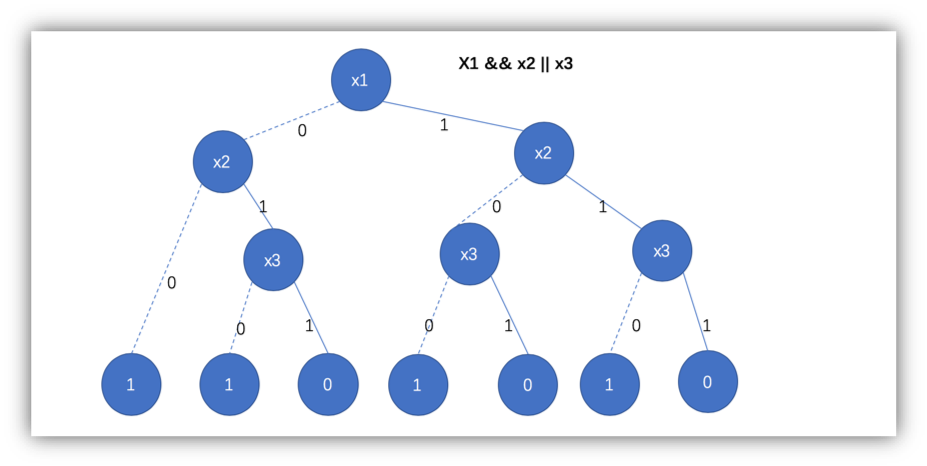1986年R.E. Bryant()给出了BDD的一个有效的图表示及其相关的算法，根据维基百科的介绍，Bryant的这篇论文在科学文献索引CiteSeerX里计算机分类里的被引用率第一的文章。Bryant在2009年获得了被认为是电子设计自动化界的诺贝尔奖的菲尔·卡夫曼奖。

Bryant认为BDD的构造过程中，变量的顺序是关键的，例如上面的例子，我们使用x3->x2->x1的顺序，得到的BDD如下：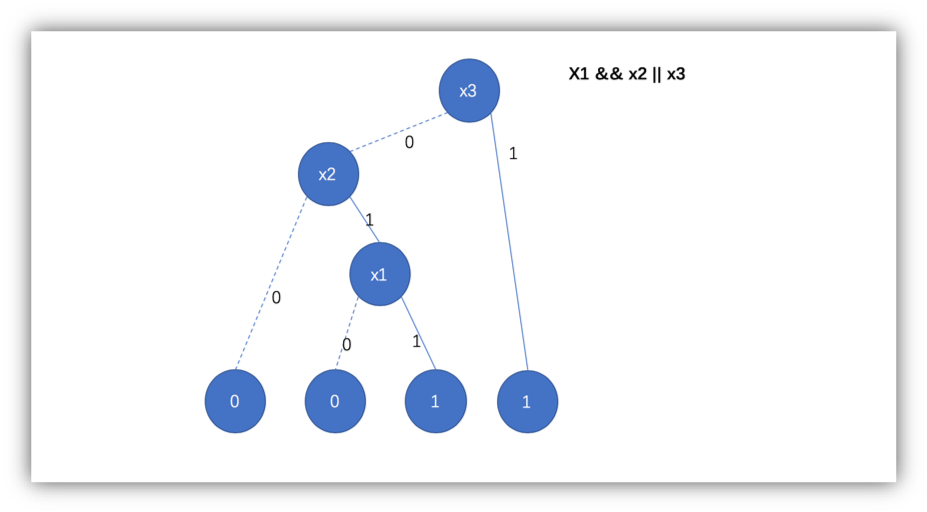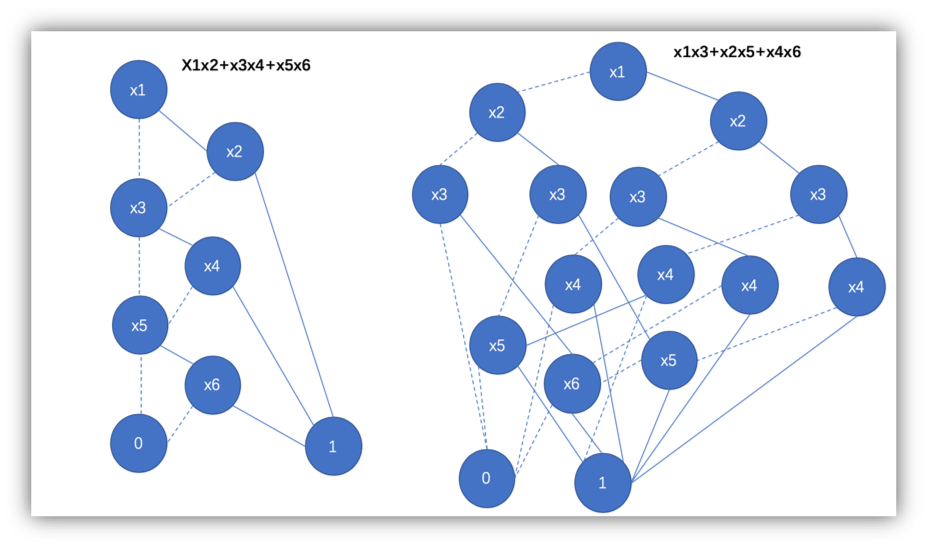• 存在冗余的节点，例如终端节点(terminal)只有两个值0和1，但是重复了多次。
• 存在冗余子图，例如两个非叶子结点(non-terminal)后面的子图是一样的结构。

• k-ary布尔函数的输入变量是x1,x2,...,xk;
• 终端节点0编号为0，终端节点1编号为1；
• 一个非终端节点u，定义low(u)为u节点取0时的输出节点；
• 一个非终端节点u，定义high(u)为u节点取1时的输出节点；• 一个终端节点u，如果是0节点，则定义var(u)=0；如果是1节点，则定义var(u)=1;
• 一个非终端节点u，定义var(u)=i，如果u上面的判定变量是xi;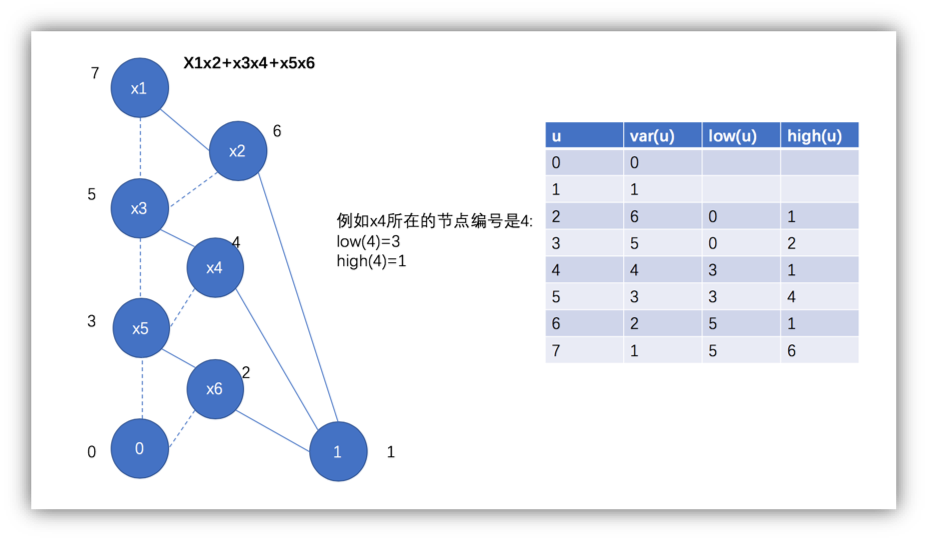• 消除冗余的终端节点(Remove duplicate terminals)
• 在BDD里面，终端节点就最多只能有两个，0和1.
• 消除冗余的非终端节点(Remove duplicate nonterminals)
• 如果var(u)=var(v)，并且low(u)=low(v),high(u)=high(v)，就消除v，v的输入都定向到u
• 消除冗余的测试(Remove redundant tests)
• 如果low(u)=high(u)，那么消除u和high(u)，输入都定向到low(u)

• 只有1个或者2个终端节点，0和1
• 非终端节点只有2个子节点low(u)和high(u)
• 每个节点上可以定义一个变量函数var(u),var(u)等于u上判定变量xi的下标i
• 从根节点到终端节点的路径上的变量顺序都保持x1<x2<...<xk的顺序
• 唯一性(uniqueness)。不存在两个节点u和v使得var(u)=var(v), low(u)=low(v), high(u)=high(v)
• 非冗余测试性(non-redenced-test)。不存在节点u使得low(u)=low(v)。

• $t^0$=0
• $t^1$=1
• $t^u=var(u)->t^{high(u)},t^{low(u)}$，如果var(u)对应的变量取值1，则$t^u=t^{high(u)}$，否则$t^u=t^{low(u)}$

• 使用数学归纳法，证明递归函数$f^u$的上述典范性质。

## 0x05 BDD算法和工具包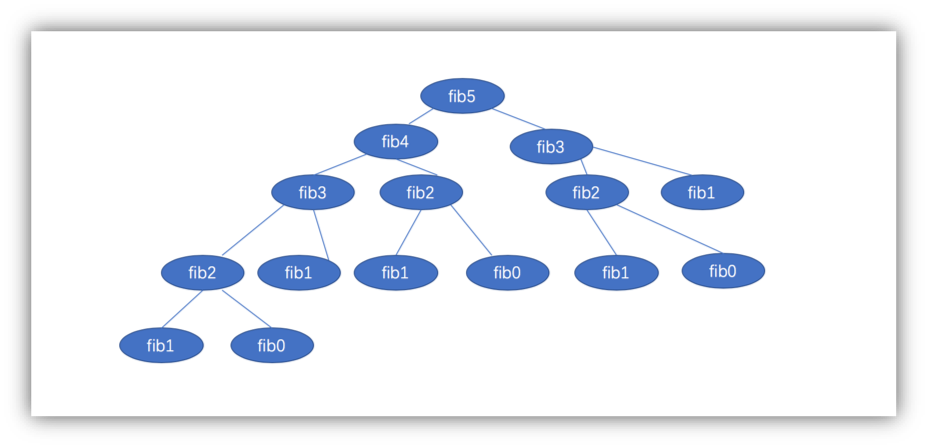• init(T),初始化的时候只有节点0和1及其对应的三元组
• var(u),low(u),high(u)，计算u的三元组

• init(H)，空表
• member(H,i,l,h)，检查(i,l,h)是不是已经在表里面
• lookup(H,i,l,h), 找到(i,l,h)对应的u
• insert(H,i,l,h,u)，插入(i,l,h)对u的映射

function MK_T_H(i,l,h){
if(l==h){
return l;
}else if(member(H,i,l,h)){
return lookup(H,i,l,h)
}else{
insert(H,i,l,h,u);
return u;
}
}

function BUILD_T_H(t,n){
function build(t,i,n){
if(i>n){
return t?1:0;
}else{
l = build(t[1/x1],i+1,n);
h = build(t[0/x1],i+1,n);

return MK_T_H(i,l,h);
}
}
return build(t,1,n);
}

• Apply,在节点u上应用某个操作。
• Restrict，限定某些变量为常数的情况下的BDD。
• SatCount，计算所有从根节点到终端节点1的不同路径数。给定ROBDD的情况下，计算可满足性是容易的。
• AnyStat，找出一条从根节点到终端节点1的路径。
• AllStat，找出所有的从根节点到终端节点1的路径。
• Simplify，给定节点d和u，如果存在u'使得$t^d \wedge t^u$ = $t^d \wedge t^{u'}$，那么消除u'.

ROBDD有各种语言实现的工具包，这个github仓库上收集了所有语言的BDD工具库：
https://github.com/johnyf/tool_lists/blob/master/bdd.md

## 0x05 BDD的应用场景

ROBDD在下面的很多场合中应用：

• 8皇后问题，由于8皇后问题可以表示为析取式(DNF)，因此可以用ROBDD计算。
• 组合电路(Combinational Circunit)的正确性验证.
• 组合电路的等价性，ROBDD唯一表示布尔函数为此提供了方案。
• 形式验证(formal verification)中判定一个系统是否拥有性质P，实际上是解决可满足性问题。
• 最优化问题的解决
• 编程语言上的应用()
• ......

## 0x06 参考

 [Aker78] S. B. Akers, "Binary Decision Diagrams", IEEE Transactions on Computers, vol. c-27, no. 6, June 1978. https://www.computer.org/csdl/trans/tc/1978/06/01675141.pdf
 [Brya86] R.E. Bryant, "Graph-Based Algorithms for Boolean Function Manipulation", IEEE Transactions on Computers, vol. c-35, no.8, Aug. 1986. https://www.cs.cmu.edu/~bryant/pubdir/ieeetc86.pdf
 wiki:Boolen function
[5.a] wiki:Propositional formula
[5.c] wiki:Boolan expression
 wiki:Binary decision diagram
 wiki:Boolean satisfiability problem
 wiki:Propositional directed acyclic graph
 wiki:Negation normal form
 wiki:Circuit complexity
[11.a] wiki:Boolean circuit
[11.b] wiki:Functional completeness
 cs.cmu.edu(15122):Binary Decision Diagrams
 cmi.ac.in:An Introduction to Binary Decision Diagrams
 BuDDy - A Binary Decision Diagram Package
 cs.cmu.edu(15817):Model Checking I:Binary Decision Diagrams
 algorithm on Binary Decision Diagrams
 cs.ox.ac.uk: An Introduction to Binary Decision Diagrams
 eecs.berkeley.edu:Binary Decision Diagrams
 csail.mit.edu:Using Datalog with Binary Decision Diagrams for Program Analysis(bddbddb)
 Modern SAT solvers: fast, neat and underused

posted @ 2018-08-07 17:38 ffl 阅读(...) 评论(...) 编辑 收藏